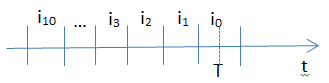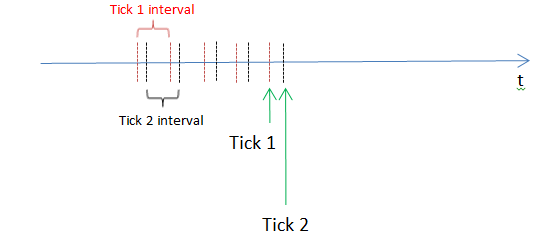# Closing (High, Low) price calculation13

Hi,

I have the following question regarding the calculation of technical indicators.

Technical Indicators (such as EMA) usually rely on prices calculated for a set of given time periods. For example, we take a time interval of 5 mins. We then calculate High, Low, Open, Close (HLOC) prices for this interval. Say, our EMA operates on the last 10 intervals, this means we have to calculate HLOC for 10 intervals into the past.

My question relates to the way HLOC for technical indicators are calculated (and Closing price in particular for this case, as EMA usually operates on Closing prices).

Let’s assume we are now at the time “T” inside the time interval i 0 .At this point in time we have 3 options to calculate the closing prices.

1) We simply use the Closing prices from previous intervals i 1, i 2, i 3 and so on up to and including an interval i 10 . I.e. at the time “T” and during an entire interval i 0 the value of Closing price and therefore of an EMA will remain the same.

2 ) Throughout the interval i 0 we constantly update the Closing price (along with High and Low) for every tick. For the remaining 9 data points needed to calculate our EMA we use previously calculated values from intervals i 1 to i 9 .

3) With every tick we move the boundaries of time intervals. I.e. instead of having fixed time interval boundaries at every tick we move them and recalculate HLOC for all 10 intervals into the past.My question is then: which one of these methods is an industry standard? For example, I have noticed that MetaTrader 4 constantly updates HLOC for the last time interval, thus probably employing the 2 nd method.

Thank you,

Konstantin6636

kostyabkg :

Hi,

I have the following question regarding the calculation of technical indicators.

My question is then: which one of these methods is an industry standard? For example, I have noticed that MetaTrader 4 constantly updates HLOC for the last time interval, thus probably employing the 2 nd method.

Thank you,

Konstantin

Don't make it more difficult then needed....

 PRICE_CLOSE 0 Close price. PRICE_OPEN 1 Open price. PRICE_HIGH 2 High price. PRICE_LOW 3 Low price. PRICE_MEDIAN 4 Median price, (high+low)/2. PRICE_TYPICAL 5 Typical price, (high+low+close)/3. PRICE_WEIGHTED 6 Weighted close price, (high+low+close+close)/4.

All those prices can change if you take the value at latest bar except PRICE_OPEN

The Openprice of an bar is never changing

So why not do take for emaperiods the openprices of the bar instead of closeprice..

So you can do take the value of bar 1 at first tick if you don't want to have the calculation changed

or you calculate the EMA Price_Open at bar 013

Hi deVries,

Thank you for your reply. However my question is a bit more generic: if you take the trading community as a whole - what would be the "de facto" method for calculating an indicator? I am a PhD student and doing some research on this. Please refer to the 3 points in my first post.

Thanks!

Konstantin6636

Every Period() stays the same a formed candle is not changing

there is no tick1 interval there are periods of time interval

if you calculate over periods then every timeperiod has its own high low openprice and closeprice

depends what calculation method you want to use if you need high open low and/or close

over the calculation we don't change timeperiods

so no running periods...

time you have to see in mq4 programs as number of seconds elapsed from 00:00 January 1, 1970.

if you have 5 minute period then every new 5 min period where is trading done you will find a new bar

it might be for example you have 1 or more then 5000 ticks there will be a high low opening and closing price92

i want to make one indicator which plot lines for last year open high low close ... 4 lines in the chart and quarter period lines for open high low close... so total 8 lines..

kindly help on thisModerator
20422

ramzam:

Do not double post!

Your other post has been deleted.20214

ramzam: kindly help on this
1. Don't double post.